## Tuesday, November 30, 2010

### Duly Quoted

On "overfitted" models:

J.Bertrand said it this way: 'Give me four parameters and I shall describe an elephant; with five, it will wave its trunk.

--L. Le Cam

first page here
behind a firewall, sorry :(
do the jstor thing if you can..

### Duly quoted

If a man is offered a fact which goes against his instincts, he will scrutinize it closely, and unless the evidence is overwhelming, he will refuse to believe it. If, on the other hand, he is offered something which affords a reason for acting in accordance to his instincts, he will accept it even on the slightest evidence.

--Bertrand Russell
cited here with many others

## Monday, November 29, 2010

### Notes on Phylip file format

The file format for this well-known suite of programs (here) is described in the main documentation file (here) in the section labeled Data file format. It is, as Prof. Felsenstein says, a "rather stereotyped input and output format," and I thought I would explore it a bit to reinforce my understanding of some of the idiosynchrasies rules that weren't completely clear to me at first. I tried to do this by running the program I used for testing (`protdist`) as a Unix process, as described previously (here).

The most important thing I learned is that for some errors, the process does not return an error code, it returns 0. So you can't use the faster method to test files for correct formatting. You have to get a special lime-green terminal window up and type the commands in yourself.

Another iimportant rule is that in sequential ('aligned') format (all of seq 1 followed by all of seq 2 etc), this program at least, does not allow blank lines between the sequences. The error is:

 `ERROR: end-of-line or end-of-file in the middle of species name for species 2`

Yet, as mentioned, when run as a process this does not return an error. Other details:

 `The number of sequences and number of characters on line 1:Alignment at the left margin with a single space between worksAnother character (';') between does not, though the process returns 0The number of sequences must be correct.The number of chars can be less but not more than are actually presentAs the docs say, the sequences must have names.The names must have <=10 chars, and be filled out to 10 with spaces.If a name is 10 chars, the sequence should start immediately, no space.Spaces within sequences, like groups of 10, is optionalSequences don't have to start at left hand margin after the first line.Species name cannot be on a separate line.A blank line between groups of seqs is optional in interleaved format.Blank line is not allowed in aligned (one whole sequence then another)`

## Sunday, November 28, 2010

### Heat map meets RNA secondary structure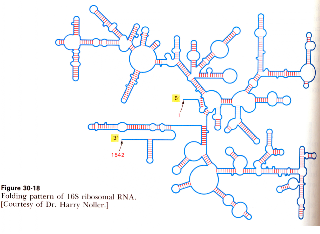I have seen somewhere a combination heat map and secondary structure plot of the bacterial 16S rRNA gene. Something like the graphic above but with colors that show the extent of conservation at each position. I think it's probably what is referenced in PMID 8811093, except that the server listed in the reference seems to be offline, and what's in the paper doesn't look as great as what I remember.

So that's our project for today. The first thing I did was go to a page at NCBI that list all the completed bacterial genomes and gives a link to pages with their RNA or protein products. I'm not actually sure where this page lives any more, I got the link from an old post. :)

I got the sequence of rrnC from E. coli MG1655 and then I shortened the title and cut out everything beyond nt 560:

 `>EcoliAAATTGAAGAGTTTGATCATGGCTCAGATTGAACGCTGGCGGCAGGCCTAACACATGCAAGTCGAACGGTAACAGGAAACAGCTTGCTGTTTCGCTGACGAGTGGCGGACGGGTGAGTAATGTCTGGGAAACTGCCTGATGGAGGGGGATAACTACTGGAAACGGTAGCTAATACCGCATAACGTCGCAAGACCAAAGAGGGGGACCTTCGGGCCTCTTGCCATCAGATGTGCCCAGATGGGATTAGCTAGTAGGTGGGGTAACGGCTCACCTAGGCGACGATCCCTAGCTGGTCTGAGAGGATGACCAGCCACACTGGAACTGAGACACGGTCCAGACTCCTACGGGAGGCAGCAGTGGGGAATATTGCACAATGGGCGCAAGCCTGATGCAGCCATGCCGCGTGTATGAAGAAGGCCTTCGGGTTGTAAAGTACTTTCAGCGGGGAGGAAGGGAGTAAAGTTAATACCTTTGCTCATTGACGTTACCCGCAGAAGAAGCACCGGCTAACTCCGTGCCAGCAGCCGCGGTAATACGGAGGGTGCAAGCGTTAATCGGAA`

I used the Vienna RNA server to fold it, and recovered a heat map version as an eps file. You can check the predicted structure against the scan above, it looks pretty good. The eps file lists the colors in terms of a heat map. But the values are for base-pair probabilities, not what I want. Luckily, the colors are encoded as values between 0 and 1, one on each line from 876 to 1435 (= 560).

I grabbed 100 of my personal database sequences at random, added the E. coli sequence and used MUSCLE to make an alignment. Then I wrote two simple scripts. One gets the counts for each position that is present in the E. coli sequence and writes them to disk. The second one assigns colors to the values based on whatever filter I put in it, the one here is:

 `steps = [ 0.97, 0.9, 0.8, 0.7, 0.6, 0.5, 0.4 ]colors = [ 1.0, 0.95, 0.8, 0.6, 0.45, 0.3, 0.15 ]`

For each set of counts, we get the maximum, then at each step if we're over the step we return the color.

That script also modifes the eps file. Afterwards, I just double-click to open the eps file in Preview. It looks great! I added the color bar from the original version by hand. Zipped folder with project files is here. Since the eps file contains the layout position for each nucleotide, we could get more sophisticated and do the whole plot ourselves.

Note: no error checking at all yet. Hope there's no whoppers...
[ UPDATE: Notice the co-variation among paired bases, they tend to have the same color. Awesome! ]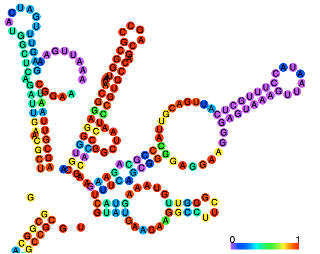### The case against intelligent designI always make a point of telling my students about the fatal problem that type III secretion poses for Michael Behe's use of the bacterial flagellum as an example of "irreducible complexity" (discussion here).

Today I came across a paper (here) by Joe Felsenstein that was new to me, which deals with the other intellectual force behind Intelligent Design, William Dembski. In classic Felsenstein style, he restates the arguments in a way that starkly reveals their problems. His analogies are simply fantastic. It's very well written, and a must read for anyone interested in bioinformatics.

### Golden oldies: Sarich and WilsonI introduced the topic of molecular phylogenetics to students by looking at some classic papers in the field. I want to summarize a few of those (by Vincent Sarich and Allan Wilson) here. This will give me a chance to show some nice pictures like the montage above.

The images are from wikipedia. Top-left is Colobus, an "old world" monkey. On the right is Cebus, a "new world" monkey. And bottom left is a Lemur. Just for fun, here is Vincent Sarich himself.I found the image here.

I'm sure you know the story, but just to remind everyone, there is a huge amount of geological evidence that the American continents have moved with respect to (particularly) the African continent. I'm not sure of current estimates as to the date of separation but one reference says about the end of the Cretaceous period (65 Mya). (There is apparently an argument about whether a land bridge remained for an extended period of time). Here is what the world is thought to have looked like a little while before that: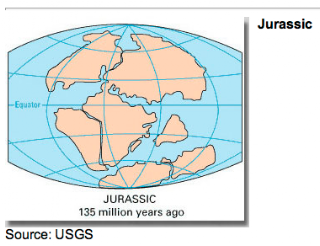found it here, with a USGS credit

Separation enforced separate evolution of lineages giving rise to modern old and new world monkeys, with the hominid lineage separating from that of the old world monkeys at a substantially later date. Here is a figure illustrating the point from the 1967 PNAS paper (Sarich and Wilson 1967 PMID 4962458).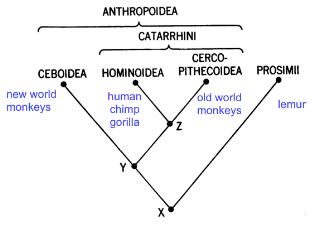It's a classic example (perhaps the classic example) of allopatric speciation, with speciation happening after geographical isolation by barrier formation (the Atlantic Ocean). Image from here:In a series of papers, Sarich and Wilson described one of the first "molecular" phylogenetic studies. To be clear, it is primarily based on a relatively complex immunological test, but antibodies are molecules even if this is not a sequence-based method. Our goal for today is to understand the test, and look at some of the data from the first paper (Sarich and Wilson 1966 PMID 4958934).

The assay is a micro complement-fixation assay, which is slightly funny since the assay volume is 7 ml---massive by modern standards. It's an immunological assay involving antibody and antigens. The antibody in this case is rabbit antiserum from animals immunized with purified human serum albumin. The antiserum is diluted (between 1:1000 and and 1:11000) and incubated with varying amounts of antigen---serum albumin. In the first part of the paper they use purified human protein, and then later they use serum containing albumin from a variety of primate species.

It isn't clear to me what fraction of the 7 ml is antiserum, but they say a curve (8 points) requires 1 ul of antiserum, so it isn't very much.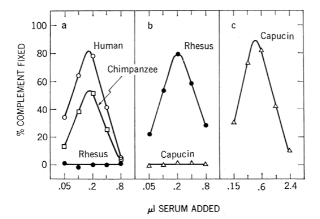The test used here is a complement fixation assay. The readout at the end of the test is lysis of sheep red blood cells (RBCs) by the membrane-busting action of complement, after the RBCs have been "sensitized" by pre-treatment with Ab (a different Ab, directed against surface antigens of the RBCs). Lysis releases soluble hemoglobin into the test tube, which can be quantified using a spectrophotometer.

The more lysis observed, the more active complement was present. If the rabbit antibody to human serum albumin finds available antigen and binds to it, that activated antibody can bind to complement, which removes it from the system, so that it will no longer promote lysis of the RBCs. In contrast, antibody molecules alone, or antigen (human serum albumin) molecules alone, are not able to bind to complement. Complement "fixation" (and thus, antibody-antigen complex formation) is measured by a decrease in the observed lysis of RBCs. So far, so good.

Now, in typical immunological fashion, the reaction between antibody and antigen depends on both the concentration and the relative amounts of the two components. The dependence on relative amounts arises because antibodies are multivalent (divalent for IgG), and the antigen used here has multiple epitopes to which the many different kinds of antibodies in the antiserum may bind as well. In antibody-excess (left part of the curve; top of the figure below) most complexes are of single antigen molecules bound to multiple antibodies; in antigen-excess (right part of the curve, bottom panel of figure) most complexes are of single antibodies bound to multiple antigen molecules.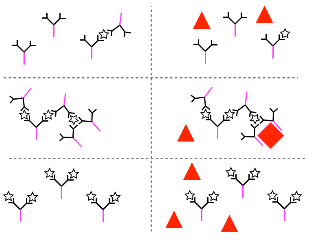Aggregates containing many molecules of each type are formed when just the right ratio of antibody to antigen is present. In a "macro" assay, this would be observed as a precipitation, observable by eye. Here, for some reason only the networks are efficient at "fixing" complement, that is, removing it from the system. The reason seems to be that multiple Fc chains (the magenta rods in the figure) need to cooperate to "fix" the complement. I've shown the free complement as red triangles, and the bound complement as red squares, because a conformation change occurs upon binding, and the complement component (C1q according to this) is sequestered (nice animation here). I'm a little uncertain as why this disables the complement activity against the sheep RBCs, since the point of binding to the antibody is to do something, but that seems to be the story.

In any case, the use of complement gives a very large amplification of the signal from each antibody-antigen complex, because loss of each "bit" of complement by binding to our complexes causes a large loss of the RBC lysis signal. (Sound complicated: it is! Is it linear with evolutionary distance? Who knows?)

The sharpness of the peaks has been accentuated by a logarithmic transformation of the x-axis.

Not surprisingly, the reaction of these rabbit antisera to human serum albumin is very strong, and can be observed at a substantial dilution (1:11000). At this highest dilution only human and chimp proteins give a visible signal in the assay. Quite small adjustments (panel b antiserum is 2.5 x concentrated, panel c is 4.4 x), result in observation of reactivity for the purified serum albumins from other species.

My inclination might have been to compare the position of the peak on the x-axis with a purified standard antigen to that observed with the serum samples (below), which seems like it would be subject to a fair amount of error. But what these authors do is focus on the height of the peak. The difference between peak heights for human and chimp, say, is easily measured.
The chimpanzee curve has a peak whose height is 67 percent as great as that given by HSA.

The analysis proceeds by plotting the peak height observed for different dilutions of antiserum. (Log-transformed) values give a straight line. The difference between antigens is summarized by taking the difference in dilution which yields 50% fixation. Guesstimating from the figure 50% fixation occurs for human at about 1:9500, for Rhesus at about 1:4000, so the "index of dissimilarity or immunological distance" between them is 9500/4000, approximately 2.4.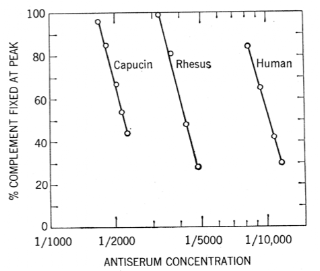They also showed that reciprocal tests (anti-human SA with chimp SA compared to anti-chimp SA with human SA) gave comparable results. Then, the real interest was to extend the test to a number of different species, which was done by using not purified antigen, but antigen in serum samples.

The ID observed using rabbit antiserum to human serum albumin combined with serum from various species was:

`human 1.0chimp 1.14gorilla 1.09old world monkeys 2.23-2.65new world monkeys 4.2-5.0 *prosimians 7.6-18* one exception`

The species studied were:

 `Hominoids:Homo sapiensPan troglodytesGorilla gorillaPongo pygmaeusHylobates larSymphalangus syndactylusCercopithecoidea (Old World monkeys):Macaca mulatta (Rhesus)Papio papioCercocebus galeritusCercopithecus aethiopsColobus polykomosPresbytis entellusCeboidea (New World monkeys):Aotes trivirgatusAteles geoffroyi (spider monkey)Saimiri capucinusCallicebus torquatusProsimii (Prosimians):Tarsius spectrumGalago crassicaudatusNycticebus coucangLemur fulvusTupaia glisNon-primates:Bos taurusSus scrofa`

### Plotting the transition bias in evolutionI saw this graphic in Page & Holmes and thought it was really nice (kind of reminds me of the old letter counts script in the analysis of Ulysses (here), and I thought it would be fun to try to recreate it. Today we're going to do the left-hand plot, the one that originates from the alignment of the human and chimpanzee mitochondrial DNA sequences.

First we need the sequences of the human and chimpanzee mitochondrial genomes. Go to the NCBI page and get: Homo sapiens (NC_012920 - 16569 nt) and Pan troglodytes (NC_001643 - 16554 nt).

For reasons that surpass understanding, the two are not in synch. This can be seen from a pairwise BLAST, or by looking at the gene layouts after following the links from the main mitochondrial genomes page:

 ``Homo ND1 3307-4262Pan ND1 2725-3681``

Homo looks like it has about 582 nt extra on the front side, which matches reasonably well with the extra '-' inserted to the Pan sequence observed from a preliminary alignment run with MUSCLE. I saved that run of '-' to a file and counted them:

 `>>> FH = open('extra.txt','r')>>> data = FH.read()>>> data.count('-')576`

So then I looked at the very beginning of the Pan sequence in the alignment, and searched for the same 10-mer sequence (GTTTATGTAG) in human at about the right place. To check, I pasted the preceeding human sequence into a file and measured its length:

 `>>> FH = open('extra.txt','r')>>> data = FH.read()>>> data = data.replace('\n','')>>> data'GATCACAGGTCTATCA...>>> len(data)576`

Looks good. Just cut that from the front of human and paste it at the end of the original sequence. Then rerun the alignment:

 `\$ muscle -in homo.pan.mito.txt -out align.txt`

No extra '-' to be seen on the front or back of the sequence after alignment. Overall, there are a few gaps, not many.

 `>>> FH = open('align.txt','r')>>> data = FH.read()>>> homo,pan = data.split('>Pan')>>> homo.count('-')6>>> pan.count('-')21`

The next step is to count the number and kind of the differences. We save a count for each pair in a dict. In the run shown here, I consolidated 'A','C' and 'C','A' (for example) as one entry. However, I used the actual values (that is A-C different than C-A) for the plot.

 `('-', 'A') 6('-', 'C') 9('-', 'G') 2('-', 'N') 1('-', 'T') 9('A', 'A') 4871('A', 'C') 63('A', 'G') 420('A', 'T') 47('C', 'C') 4645('C', 'G') 14('C', 'T') 904('G', 'G') 1930('G', 'T') 6('T', 'T') 3648-------------------- 16575 total 15094 identical 1324 transition 130 transversion 27 gap0.0879TC.CT.CT.TC.TC.TC.AG.CT.CT.AG.TC.CT.CT.CT.GA.TC.CT.TC.CT.GAAC.TA.AC.AC.AT.CA.AC.TA.AT.GC.CA.CA.TA.GT.AT.TA.CA.TA.AT.TA`

I printed a few of the transitions and transversions as a sanity check.

The plot uses `scatter` as we've done many times before, and I added the labels by hand. The values are log-scaled to make the smaller ones more visible. The file is on Dropbox (here).

We don't really match the original very well. It shows either C-T or T-C as larger than C-C. The plot doesn't show that, and neither do the actual counts. I don't think it can be a problem with the alignment, since the sequences are so close. Guess I'll have to track down the original reference to know why.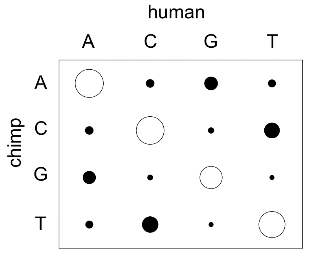## Saturday, November 27, 2010

### Duly quoted: Steven Chusource

The other issue is that the ideas are complex. Now, if you step back and if you spend some serious time thinking about it, the kernel of the ideas are clearly not complex. The essence of an idea is what we try to work with most. As a professor with my graduate students, we would read a paper and look at the paper and say, "What's the essence of the idea? What's something new? Forget about the equations. Forget about the complicated argument and try to identify the kernel of the idea."

--Steven Chu

### Phylogenetic tree surgery in Python: NNI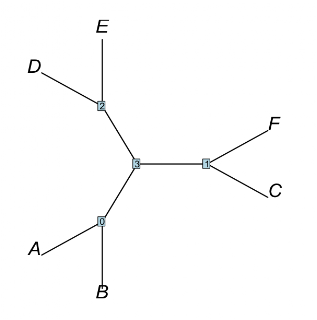I implemented this relatively simple type of tree surgery in Python. The example is the one we talked about last time (here). The plot of the result is above, and for reference, the original tree is below: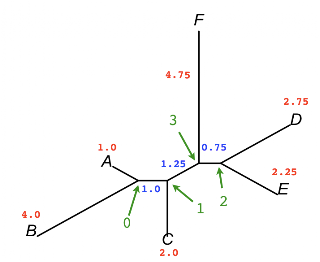The output shows the logic:

 `0 ['A', 'B', '1']1 ['C', '0', '3']2 ['E', 'D', '3']3 ['F', '1', '2']--------------------internal nodes: 1 3exchanging: C 21 ['0', '2', '3']3 ['C', 'F', '1']fixing e2: 2before: ['E', 'D', '3']after: ['D', 'E', '1']--------------------0 ['A', 'B', '1']1 ['0', '2', '3']2 ['D', 'E', '1']3 ['C', 'F', '1']`

The first section is the initial tree. The middle part documents the changes we're making and the last prints out the result. I parsed the tree using our previous code, and plotted it using R's ape. I also added the code (`nni.py`) to the zipped files for the project. It's been fun, but I probably won't do the others for a while, if at all. I'm ready for new challenges.

### Phylogenetic tree surgery 1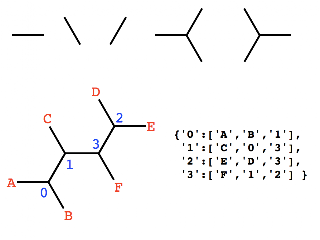One of the biggest problems in phylogenetic analysis is the very large number of possible trees. I don't think I've posted about that yet, but you probably appreciate that for N species the number of trees is approximately 10N. (Speaking very roughly). For 25 species we're talking more than a mole of trees. That's a lot!

One consequence is that we can never evaluate all the possible trees for any problem of significant size. Furthermore, if we have a tree that we like (it's the one with the maximum likelihood that we've seen so far), it becomes important to look at its near neighbors in "tree space" and see if they are any better. Felsenstein has a great discussion of this in Chapter 4.

I want to study a bit about the basic methods of tree surgery. My hunch is that representing trees in the way that was introduced previously (here), as lists of connections to each internal node, will make this pretty easy. My goal in this post is simply to present one example of each of the methods that I know about, and show the reorganization of the list that results. The examples are shown in the figures before, which were constructed in Keynote. We'll explore these in future posts, as time permits. The plan is to develop rules to emulate the types of list reorganization observed here, and see if the structure of the resulting trees can be explained by that type of surgery.

In each example, the list entries that change are highlighted.

In NNI we pick a branch, and rearrange the quartet of clades to form a new tree. There are two possible rearrangements. Here is one of them for the 1-3 branch.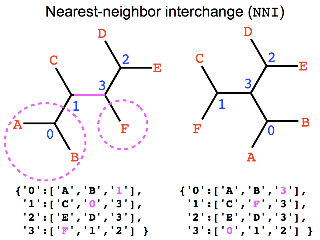In SPR we imagine cutting in the middle of one branch, and then connecting one of the pieces to the middle of a second branch in the other piece. I had to relabel the internal nodes for this one, I did it in a way that makes the changes to the list easiest.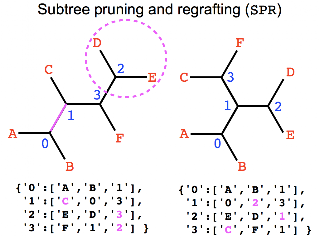In TBR we cut a branch and dissolve the stubs, then pick one branch in each of the smaller pieces, and connect there.### Neighbor-joining in Python: doing the plot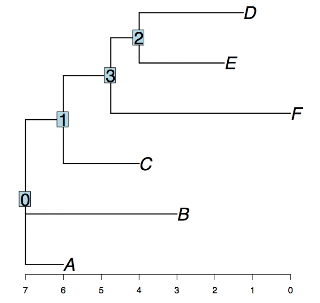As discussed last time, I'm using Python lists to hold the information for each node on a phylogenetic tree. This seems as if it will have significant advantages, especially when it comes to tree "surgery." I'll leave that for another post. What I want to do today is actually draw a tree rooted at each of the internal nodes. Here is our example:

 `example_tree = { '0':['A','B','1'], '1':['C','0','3'], '2':['E','D','3'], '3':['F','1','2'] }`

Code to traverse the tree was shown last time (here). I've modified it slightly to use a dictionary. Previously we had this representation for each node and its parent (in the traversal from a particular root):

 `A:0, C:1, B:0, E:2, D:2, F:3, 1:root, 0:1, 3:1, 2:3`

Now these keys and values are in a dict. The results of the traversals from each internal node as root are:

 `A:0, C:1, B:0, E:2, D:2, F:3, 1:1, 0:1, 3:1, 2:3A:0, C:1, B:0, E:2, D:2, F:3, 1:0, 0:0, 3:1, 2:3A:0, C:1, B:0, E:2, D:2, F:3, 1:3, 0:1, 3:3, 2:3A:0, C:1, B:0, E:2, D:2, F:3, 1:3, 0:1, 3:2, 2:2`

The root node is now marked by having itself as its "parent."

The drawing code works by building a `repr` (representation) for each node from the farthest tips working up to the root. The method was inspired by PyCogent's `ancestors()` function, which returns a list of ancestors (naturally enough). The output with debug enabled looks like this:

 `ancestorsE ['2', '3', '1', '0']D ['2', '3', '1', '0']F ['3', '1', '0']2 ['3', '1', '0']C ['1', '0']3 ['1', '0']A ['0']B ['0']1 ['0']0 []`

I simply sort on the length (largest first) and work in that order. So E's `repr` is just 'E'. When we process the first i_node (2), it's `repr` will be `(E,D)2`. If we're using branch lengths, it will be: `(E:2.25,D:2.75)2`. The distance data is in a separate dictionary:

 `dist = { ('A','0'):1.0, ('B','0'):4.0, ('C','1'):2.0, ('D','2'):2.75, ('E','2'):2.25, ('F','3'):4.75, ('0','1'):1.0, ('1','3'):1.25, ('2','3'):0.75 }`

Here's the resulting Newick tree:

 `(A:1.00,B:4.00,(C:2.00,(F:4.75,(E:2.25,D:2.75)2:0.75)3:1.25)1:1.00)0;`

One of the plots is shown at the top, a second one is below. Although I had it working before, be advised there's a bug somewhere that blows up R when I try to plot the tree as 'unrooted.' So now my to_do list includes the challenge of fixing that. [UPDATE: It seems to only happen when calling R from Python using RPy---probably related to the use of a Python keyword type as an argument to the R function. ] Zipped project files are here. And a final note, I've included the upgma code from before. However, we processed the nodes with a different algorithm there, so the drawing code is different, and I haven't tried to convert it to the new method yet.## Friday, November 26, 2010

### Tree representation and one kind of traversalShown above is the tree we generated using the neighbor-joining method (here and here). I've annotated the plot from ape to label the internal nodes and show all the branch lengths. The internal node labels were assigned in the same order as they were clustered to build the tree. I didn't mention it before, but it's important to remember that this is an unrooted tree, and that's why it's drawn as it is, rather than with all the branches laid out horizontally, as in the UPGMA example (here).

I want to spend some time on trees in general, without worrying about how they were built. One goal is to understand the different modes of tree traversal (preorder and postorder and so on). Another is to explore different internal representations for trees in Python code, and conversions to and back from Newick format (and possibly XML format). In addition, I'd like to understand what it takes (in code) to "root" a tree, and how to implement various methods for tree "surgery" like "tree pruning and re-grafting" (e.g. here), and what the implications are for the Newick structure, which I find a bit hard to grasp.

In today's (very simple) example we're just going to try to visit all the nodes. The first thing is to decide on a way to represent the tree. The basic structure is naturally a dictionary, because we want to be able to "look up" the data for any individual node quickly. In a simplifying (and liberating) decision we're going to use a simple Python list to hold that data. One advantage of this is that we could accommodate star topologies easily. (And we could remember distance information by using a tuple of two lists, the names in one and distances in the second). Although a string would also do here, in general it won't work because we want to allow labels with `len(label) > 1`.

Only the internal nodes are in the dict; it looks like this:

 `tree_dict = { '0':['A','B','1'], '1':['C','0','3'], '2':['E','D','3'], '3':['F','1','2'] } `

At this point, we have no notion of directionality. That's the liberating part. We will gain more structure as we traverse the tree.

Code to visit all the nodes naturally involves recursion, a function which processes nodes and calls itself for each of the child nodes, but returns if the current node is a tip (external). The first script (below) gives the following output, the node where we start the traversal is listed first:

 `['1', 'C', '0', 'A', 'B', '3', 'F', '2', 'E', 'D']['0', 'A', 'B', '1', 'C', '3', 'F', '2', 'E', 'D']['3', 'F', '1', 'C', '0', 'A', 'B', '2', 'E', 'D']['2', 'E', 'D', '3', 'F', '1', 'C', '0', 'A', 'B']`

There's one simple modification we can make to allow us to actually draw the tree. That is to record the relationship between two nodes as we descend into the tree. The second script is modified to do that. It prints:

 `A:0, C:1, B:0, E:2, D:2, F:3, 1:root, 0:1, 3:1, 2:3A:0, C:1, B:0, E:2, D:2, F:3, 1:0, 0:root, 3:1, 2:3A:0, C:1, B:0, E:2, D:2, F:3, 1:3, 0:1, 3:root, 2:3A:0, C:1, B:0, E:2, D:2, F:3, 1:3, 0:1, 3:2, 2:root`

Here, each value is a pair where the first name is the node, and the second is its parent. The "root" node is identified by using that label in place of a parent. Now we will be able to draw the tree, but actually doing that will involve some complexities which are better dealt with in a separate post.

`utils` contains `flatten` from here.

code listings:

 `import utilstree_dict = { '0':['A','B','1'], '1':['C','0','3'], '2':['E','D','3'], '3':['F','1','2'] } all = list(utils.flatten(tree_dict.values()))external = [e for e in all if not e in tree_dict]def descend(node,seen): seen.append(node) if node in tree_dict: for child in tree_dict[node]: if not child in seen: descend(child,seen)def traverse_tree(root): seen = list() descend(i_node,seen) print seen for i_node in tree_dict: traverse_tree(i_node)`

 `import utilstree_dict = { '0':['A','B','1'], '1':['C','0','3'], '2':['E','D','3'], '3':['F','1','2'] } all = list(utils.flatten(tree_dict.values()))external = [e for e in all if not e in tree_dict]def descend(node,caller,pD): pD[node] = caller if node in tree_dict: for child in tree_dict[node]: if not child in pD: descend(child,node,pD)def traverse_tree(root): pD = dict() descend(root,'root',pD) # might filter for k in tree_dict L = [k + ':' + pD[k] for k in pD] print ', '.join(L)for i_node in tree_dict: traverse_tree(root=i_node)`

## Thursday, November 25, 2010

### Threat level: yellowwikimedia

I'm sure you have a lot of usernames and passwords---I know I do. My security model has four threat levels: green, yellow, orange and red.

• green: the same password for all
• yellow: a different strong password for each one; written in an encrypted text file and transferred on a thumb drive
• orange: a strong password that I remember
• red: a password on paper, in a file cabinet that I control; orange passwords also stored here

• Level red is for banking; orange for my laptop that requires a logon at boot. I allow the Keychain to store passwords for level yellow.

The problem is how to generate a lot of strong passwords. Python is great for this:

 `import sys, random, stringcL = string.letters + string.digitscL += '@#\$%&*'def go(N): L = [random.choice(cL) for i in range(N)] return ''.join(L) try: N = int(sys.argv)except IndexError, ValueError: N = 24print go(N)'''\$ python password.py 50k5YHStb@&L4OHW4NV6fGaohDLGg8nyVYbsTRyV4aIRTTb41ob1'''`

### My version of neighbor-joining in Python

I finished debugging my version of the neighbor-joining method in Python. The zipped project files are here. I also made an nj tree from the same data using PyCogent, ape, and Phylip's neighbor. Everybody agrees!

One last thing I haven't done is to write the code to assemble the tree's representation. What I actually have is a list of internal nodes with distances to their child nodes. There's always something more to do.

PyCogent:

 `edge.0 A:1.0;edge.0 B:4.0;edge.1 C:2.0;edge.2 F:4.75;root D:2.75;root E:2.25;edge.2 edge.1 1.25 edge.2 edge.0 2.25edge.1 edge.0 1.0root edge.2 0.75 root edge.1 2.0 root edge.0 3.0 ((((A:1.0,B:4.0):1.0,C:2.0):1.25,F:4.75):0.75,D:2.75,E:2.25);`

Phylip:

 `Between And Length------- --- ------ 1 B 4.00000 1 2 1.00000 2 C 2.00000 2 4 1.25000 4 3 0.75000 3 E 2.25000 3 D 2.75000 4 F 4.75000 1 A 1.00000(B:4.00000,(C:2.00000,((E:2.25000,D:2.75000):0.75000,F:4.75000):1.25000):1.00000,A:1.00000);`

ape:

 `> tr\$edge.length 2.25 2.75 0.75 1.25 1.00 1.00 4.00 2.00 4.75> tr\$edge [,1] [,2] [1,] 7 5 [2,] 7 4 [3,] 7 8 [4,] 8 9 [5,] 9 10 [6,] 10 1 [7,] 10 2 [8,] 9 3 [9,] 8 6> tr\$tip.label "A" "B" "C" "D" "E" "F"(E:2.25,D:2.75,(((A:1,B:4):1,C:2):1.25,F:4.75):0.75);`

me:

 `0 : B 4.000 A 1.000 1 : C 2.000 0 1.000 2 : E 2.250 D 2.750 3 : 1 1.250 2 0.750 F 4.75`

## Wednesday, November 24, 2010

### Checking our test data in NJ using Phylip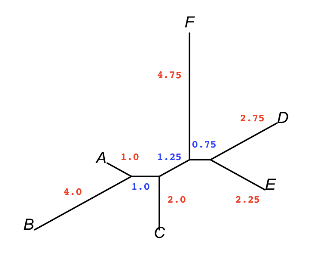I'd like to compare the results of my own neighbor-joining script to standard methods such as Phylip, ape, and PyCogent. In initial comparisons, I noticed some small differences among those methods themselves with the data from before (UPDATE: I just failed to synch the data properly). (here). I also noticed that in Phylip's input screen for neighbor, they give the option of :

 `Outgroup root? No, use as outgroup species 1Randomize input order of species? No. Use input order`

I thought it would be interesting to see if there are any differences observed in the tree if we use a different input order of species. We could use Python to rewrite the data file to change to a specific order, but as a simpler test we'll just let Phylip randomize it for us. After getting the kinks out, we do 50 reps, calling `neighbor` as a process (just like here). One wrinkle: the process returns faster than the file write from Phylip, so we have to wait a second before trying to do anything with the files ourselves.

The data for Phylip look like this (note requirement for 10 characters on each line preceeding the distances, and a count for the otus on line 1):

 ` 6A 0 5 4 7 6 8B 5 0 7 10 9 11C 4 7 0 7 6 8D 7 10 7 0 5 8E 6 9 6 5 0 8F 8 11 8 8 8 0`

As a bonus, I asked PyCogent to compare the topology of all the resulting trees, but no differences were found. And then, we plot the first one with `ape`.

 `import os, subprocess, time, sys, randomfrom cogent import LoadTreeprefix = '/Users/telliott_admin/Desktop/'controlfn = prefix + 'responses.txt'datafn = prefix + 'nj_data.phylip.txt'resultfn = prefix + 'outfile'treefn = prefix + 'outtree'def one_run(n): n = str(n) FH = open(controlfn, 'w') FH.write(datafn + '\n') FH.write('J\n') seed = random.choice(range(2,200)) if not seed % 2: seed += 1 FH.write(str(seed) + '\n') FH.write('Y\n') FH.close() cmd = 'neighbor < ' + controlfn + ' > ' cmd += 'screenout &' p = subprocess.Popen(cmd, shell=True) pid,ecode = os.waitpid(p.pid, 0) print ecode time.sleep(1) os.rename(resultfn,prefix+'results/outfile' + n + '.txt') os.rename(treefn,prefix+'results/outtree' + n + '.txt') os.remove(prefix + 'screenout')N = 50for i in range(1,N+1): one_run(i)L = list()for i in range(1,N+1): tr = LoadTree(prefix + 'results/outtree' + str(i) + '.txt') L.append(tr) for i in range(N): for j in range(N): if i == j: continue if not L[i].sameTopology(L[j]): print L[i] print L[j] else: print '.', '''R code:library(ape)setwd('Desktop')tr = read.tree('results/outtree1.txt')plot(tr,edge.width=3,cex=2,type='unrooted')axisPhylo()'''`

### Merge PDFs in OS X

I wanted to join a bunch of single page scanned tiffs into a one giant pdf. It's a chapter from a book (Shssssh!) I used Preview to save them as individual pdf files and then thought, how to merge them? I saw this hint for OS X 10.5, but it doesn't work for me on 10.6.

Then I came across another link that suggested I use pdftk (pdf toolkit). It's described here, but I got it using my new MacPorts install.

 `port search pdftksudo port install pdftk`

The usage is

 `pdftk file1.pdf file2.pdf cat output outfile.pdf`

It worked great. The only problem is the files were named: Scan1.pdf, Scan2.pdf, etc. and when sorted the order is Scan1.pdf, Scan10.pdf, etc., so they were out of order in the merge. I adopted a embarassingly inelegant solution. I shortened the names and then entered this command:

 `pdftk 0.pdf 1.pdf 2.pdf 3.pdf 4.pdf 5.pdf 6.pdf 7.pdf 8.pdf 9.pdf 10.pdf 11.pdf 12.pdf 13.pdf 14.pdf 15.pdf 16.pdf cat output Ch2.1.pdf`

Looks great!

### Five HundredAccording to `blogger`, I've put up 500 posts over about 2 1/2 years. That's a lot of posts. I've still got much more exploring to do, so I plan to continue talking about it.

In the spirit of the day, here is a picture taken earlier in the morning using Photo Booth. As you can see, I'm using only the left half of my brain!

## Tuesday, November 23, 2010

### UPGMA in Python 3: Sarich data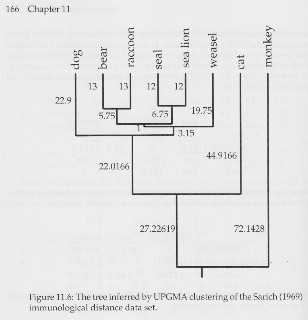In Joe Felsenstein's book, Inferring Phylogenies, he uses some data from Vincent Sarich to demonstrate UPGMA. Although I was tempted to "declare victory and go home", I decided to test my version of UPGMA with that data. And that turned up bugs in both the main `upgma` and the plotting code! So I guess the moral is: test, test, and test again.

The top figure is a scan from the book. Here is what my program plotted:It looks pretty good to me.

The data are immunological data from eight species in this order: dog, bear, raccoon, weasel, seal, sea lion, cat, monkey. The data are in this distance matrix:

 ` 0 32 48 51 50 48 98 148 32 0 26 34 29 33 84 136 48 26 0 42 44 44 92 152 51 34 42 0 44 38 86 142 50 29 44 44 0 24 89 142 48 33 44 38 24 0 90 142 98 84 92 86 89 90 0 148148 136 152 142 142 142 148 0`

If you grab the zipped files (here) and run them, you'll see a lot of diagnostic output when `debug == True`. As well as all the branch lengths.

### UPGMA in Python 2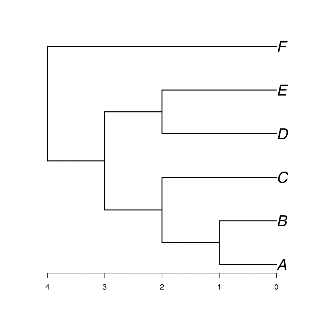Following up on the previous post (here, and an earlier post here), I wrote some code to extract the data for our UPGMA tree, and plot it using the `ape` package from R. I updated the zipped project files which are on Dropbox (here). The plot is above, it looks good to me.

To do: test on other examples! [Update: found a bug and updated again.]

### Neighbor-joiningNeighbor-joining is a standard way of constructing phylogenetic trees that is fast and does a reasonable job of recovering the correct tree in the case of unequal branch lengths. Consider this tree with vastly unequal amounts of change on the branch to B. I found this example (and the figure above) on the internet, but now I've forgotten where (sorry).

Higgs and Attwood have a description of the method that explains a bit about why the calculation is the way it is, and what guarantees it offers.

Here's the distance data:

 ` A B C D EB 5C 4 7D 7 10 7E 6 9 6 5F 8 11 8 8 8`

Step 1 is to calculate the net divergence for each OTU:

 `r(A) = 30 = 5 + 4 + 7 + 6 + 8r(B) = 42 etc.r(C) = 32r(D) = 37r(E) = 34r(F) = 43`

Step 2 is to modify the distance matrix by subtracting the value of: `(ri + rj)/(N-2)` where `N` is the number of OTUs. For example, the new value for entry 'AB' is: 5 - ((30 + 42)/4) = -13, and that is the smallest value in the whole matrix.

We choose the next two otus to cluster based on the smallest value in the modified distance matrix. In fact, we don't use this modified matrix again, so I just tested each value in turn against the low value observed so far, and kept the relevant indexes. We make a "star" tree, and then group the 2 OTUs we chose: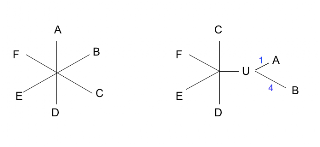Step 3. Calculate the distances of A and B from U using the data from the original distance matrix and the divergence values, as follows:

 `S(AU) = d(AB)/2 + [r(A) - r(B)/(2(N-2))] = 5/2 + (-12 / 8) = 1S(BU) = d(AB) - S(AU) = 4`

Step 4. Now calculate the distances from the new node U to the other nodes:

 `d(UC) = ( d(AC) + d(BC) - d(AB) ) / 2 = 3d(UD) = ( d(AD) + d(BD) - d(AB) ) / 2 = 6d(UE) = ( d(AE) + d(BE) - d(AB) ) / 2 = 5d(UF) = ( d(AF) + d(BF) - d(AB) ) / 2 = 7`

The result is a new distance matrix:

 ` U C D EC 3 D 6 7E 5 6 5F 7 8 8 8`

Notice that the result from this first step already looks a little more like the real tree. We repeat each of the steps until we've collapsed all the nodes. The result is an unrooted tree.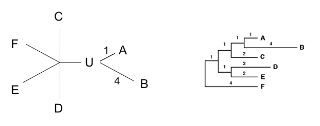R code:

 `m = c(0,5,4,7,6,8, 5,0,7,10,9,11, 4,7,0,7,6,8, 7,10,7,0,5,9, 6,9,6,5,0,8, 8,11,8,8,8,0)dim(m)=c(6,6)colnames(m)=c( 'A','B','C','D','E','F')rownames(m)=colnames(m)d = as.dist(m)library(ape)tr=nj(d)plot(tr,cex=2, tip.color=rainbow(6))axisPhylo()`

R chose a root to draw the tree that is different than in the other graphics. I forgot to fix this (with `ape.root`).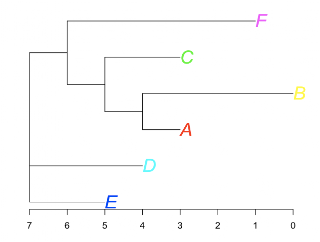[UPDATE: I'm writing a Python version of neighbor-joining, and it turned up some errors in my arithmetic. So I updated the table here.

## Monday, November 22, 2010

### UPGMA in PythonI spent a whole day working on a script to do UPGMA. (It took a lot longer than I thought it should). This first version analyzes the data from the same tree as we constructed in an earlier post (here), because it's simple.

At the end of the run, we have the correct tree, as shown by the first line in the last section of the output:

 ```(((('A', 'B'), 'C'), ('D', 'E')), 'F') A {'up': 1.0, 'parent': 'AB'} C {'up': 2.0, 'parent': 'ABC'} B {'up': 1.0, 'parent': 'AB'} E {'up': 2.0, 'parent': 'DE'} D {'up': 2.0, 'parent': 'DE'} F {'up': 4.0, 'parent': 'ABCDEF'} DE {'to_tips': 2.0, 'right': 'E', 'up': 1.0, 'parent': 'ABCDE', 'left': 'D'} ABCDE {'to_tips': 3.0, 'right': 'DE', 'up': 1.0, 'parent': 'ABCDEF', 'left': 'ABC'} ABC {'to_tips': 2.0, 'right': 'C', 'up': 1.0, 'parent': 'ABCDE', 'left': 'AB'} AB {'to_tips': 1.0, 'right': 'B', 'up': 1.0, 'parent': 'ABC', 'left': 'A'} ABCDEF {'to_tips': 4.0, 'right': 'F', 'left': 'ABCDE'}```

I struggled with trying to use the distance information to modify the string representation of the tuple on that first line, but failed. I know I can reconstruct the tree from the data for all the nodes that is in the dictionaries shown. That's for another time.

Once again, I have a better understanding through having coded an (almost) working example in Python. The files are on Dropbox (here).  [Edit:  the files are here].

## Sunday, November 21, 2010

### Clustering with hclust from PythonAs long as I have RPy working, I did a first version of a clustering script. First we do our imports and grab the definitions of R objects:

 `import sys, randomimport matplotlib.pyplot as pltimport numpy as npfrom rpy2 import robjectsimport hm_utils as hmrandom.seed(153)matrix = robjects.r['matrix']hclust = robjects.r['hclust']dist = robjects.r['dist']`

Next we generate a numpy array with random values in the set {0.5 .. 1.0}. Then we pick a few rows and mark a few of the same columns in each by changing each column's value to a random one between 0.0 .. 0.2. These show up as the light rectangles in the plot. Then we just:

 `# convert to R 'matrix' and clusterA = list(hm.flatten(A))M = matrix(A,nrow=R)d = dist(M, method='euclidean')result = hclust(d,method='average')o = result # order`

The only hard part is going back and forth between a Python array and R matrix. There's probably a better way to do it, but what I used was `flatten` from here before feeding to R, and this going the other way:

 `def to_array(M,R,C): A = np.array(list(M)) A.shape = R,C return A`

We plot a heat map using code from here. As you can see in the graphic at the top of the post, `hclust` does a reasonable job of finding the structure we created. Rather than list the code, I put a zipped folder with the files on Dropbox here.

## Saturday, November 20, 2010

### Likelihood of an evolutionary tree 2

As usual, to improve my understanding of the likelihood calculation, I wrote some code. The first module is called `likelihood.py`, and it defines three functions for processing nodes, depending on the nature of their child nodes (both external, both internal, or mixed).

It's highly commented and should be self-explanatory after the previous post (here). To keep things simple, I have not implemented variable branch lengths yet.

The problem I'm having is testing. The version that I'm actually developing has lots of statements like: `if debug: print ..`, where I've been rigid about putting the print on an indented line below the `if`. (I've stripped all of those out in the listing below). I went through the printout trying to verify the results of each calculation manually, and it's pretty exhausting. What would be nice is to have another ML program to test the output against, but without implementing the branch lengths properly, I'm not sure I can do that.

This module itself is sort of boring. I wrote another module that exercises it a lot more, but I'm not quite ready to show that yet.

The code to strip out debug statements is short and sweet:

 `data = tu.load_data(fn)data = data.strip().split('\n')def lwspace(line): return len(line) - len(line.lstrip())flag = Falsetarget = 'debug:'indent = 0rL = list() for line in data: if 'debug:' in line: assert 'if' in line flag = True indent = lwspace(line) print '*' + line continue if lwspace(line) <= indent: flag = False if flag: print '*' + line else: rL.append(line) print ' ' + line`

`likelihood.py`

 `import math# list printingdef printL(L,name=None): if name: print name pL = [str(round(n,3)).rjust(8) for n in L] N = 6 while pL: line = pL[:N] pL = pL[N:] print ''.join(line)# to start with, we'll assume equal branch lengths# construct the transition-probability matrixnt = 'ACGT'pur = 'AG'pyr = 'CT'f_same = math.log(0.95)f_transition = math.log(0.03)f_transversion = math.log(0.01)pi = math.log(0.25)def get_f(n): m,n = list(n) if m == n: return f_same if m in pur and n in pur: return f_transition if m in pyr and n in pyr: return f_transition return f_transversionk = [m+n for m in nt for n in nt]v = [get_f(n) for n in k]P = dict(zip(k,v))#---------------------------------------# three different functions # depending on types of child nodes def ext_ext(u,v): # u,v are nucleotide as char # for each possible n in their parent node # returns an array of log(p) for nu and nv rL = [P[n+u] + P[n+v] for n in nt] return rL# L = list of likelihoods in order, for internal child# v = external child nucleotide as chardef int_ext(L,v): rL = list() # for new node in {ACGT} for i,m in enumerate(nt): ep = P[m+v] # log(p) of m -> v sL = list() # each state for internal child # n is a float for j,n in enumerate(L): u = nt[j] # would multiply probs, so add logs p = P[m+u] # log(p) for next branch p += L[j] # log likelihood if that child = u p += ep # log(p) for external child sL.append(p) # will need the actual probs to add them # does this need to be done better? how? sL = [(math.e)**p for p in sL] logS = math.log(sum(sL)) rL.append(logS) return rL # both children are internaldef int_int(L1,L2,root=False): rL = list() # for new node = {ACGT} for i,n in enumerate(nt): sL = list() # each state for left child # v1 is a float for j,f1 in enumerate(L1): u = nt[j] # each state for right child # v2 is a float for k,f2 in enumerate(L2): v = nt[k] p = P[n+u] # log(p) for left branch p += f1 # log likelihood if child = u p += P[n+v] # log(p) for right branch p += f2 # log likelihood if child = v sL.append(p) # will need the actual probs to add them sL = [(math.e)**p for p in sL] logS = math.log(sum(sL)) rL.append(math.log(sum(sL))) if root: # do pi calculation rL = [e + pi for e in rL] return rL if __name__ == '__main__': #for k in sorted(P.keys()): print k, P[k] #for u in nt: #for v in nt: #ext_ext(u,v) #print '-'*40 L = [-0.1] * 4 int_ext(L,'C') print '-'*40 int_int(L,L)`

### Likelihood of an evolutionary treeCalculating the likelihood of a particular phylogenetic tree sounds complicated and it's easy to get lost in the details, but is really just a matter of bookkeeping. That's why we have computers! This example is taken from my all-time favorite Bioinformatics textbook (Higgs & Attwood).

Let's work with the tree shown above. We're considering one particular site in a sequence alignment from a set of OTUs (operational taxonomic units), where the observed nucleotides are as shown. The identities of the internal nodes are unknown and each could be any one of the four nucleotides. (I've shown the same tree several times at different places in the post for reference).

Consider first the node labeled X. In general, the branches from X to A and from X to G may be different in length. Suppose the first one is described by t1 and the second by t2.

We have a particular model of sequence evolution for this site, encapsulated in a transition-probability matrix (as discussed here), which allows us to calculate the probabilities for all 4 possibilities with no change:

 `PAA, PCC..`

and all 12 possible changes:

 `PAC, PAG, PAT..`

The identity of X isn't known, it might be any of `{A,C,G,T}`. Starting with A, then we can calculate the probabilities for the two branches as:

 `PAA(t1)PAG(t2)`

The probability of the observed data for both of these two terminal nodes with the model that X = A, is the likelihood that X = A, given the data. Namely:

 `L(X=A) = PAA(t1) PAG(t2)`

In the same way:

 `L(X=C) = PCA(t1) PCG(t2)L(X=G) = PGA(t1) PGG(t2)L(X=T) = PTA(t1) PTG(t2)`

Of course, the total probability for X = `{A,C,G,T}` is the sum:

 `Σ PNA(t1) PNG(t2)N = {A,C,G,T}`

which has to equal 1, since X must be one of the four nucleotides.

Repeated multiplication leads to various difficulties which are avoided by using logarithms. So typically we would talk about the log likelihood, for example the log of L(X=C). All of the calculations shown below as multiplications would be done in practice by adding the logarithm of each term.

### Working up the treeNow consider node Y and its branch to X, with a branch length described by t3.

We can calculate the probabilty for any choice of nucleotides (say, Y = A, X = C) in the same way as before. For this choice it is:

 `PAC(t3) PCA(t1) PCG(t2)`

It can also be written using the expression for likelihood L(X=C) as:

 `PAC(t3) L(X=C)`

Stick with probabilities for a moment.

There are 16 possible paths here. Suppose Y = M and X = N, where both M and N are in the set `{A,C,G,T}`. Then there are 16 terms like:

 `PMN(t3) PNA(t1) PNG(t2)`

This is only for the left-hand clade. We usually talk about the tree as if it were rotated with Z at the top and Y on the left, but it's easier to draw as shown. The probability for the right-hand branch from Y to G described by t4, is calculated for any particular choice of M = `{A,C,G,T}` as described in the first part:

 `PMG(t4)`

So each of our 16 terms picks up an additional factor

 `PMG(t4) PMN(t3) PNA(t1) PNG(t2)`

and the sum of the four terms involving Y=M (for a particular choice of M = A,C,G or T) is the likelihood L(Y=M).

### A node with two internal child nodesNow consider node W at the top of the tree and the branch t5 connecting it to Y. We let W assume any of the four states `{A,C,G,T}` and calculate probabilities as follows:

 `P(W=A,Y=A) = PAA(t5)P(W=A,Y=C) = PAC(t5)..`

For the t5 branch, we have three equivalent expressions:

 `P(W=A,Y=A) L(Y=A)P(W=A,Y=A) P(Y=A,X=A) L(X=A)P(W=A,Y=A) P(Y=A,X=A) P(X=A,left=A) P(X=A,right=G)`

There are 16 terms like:

 `P(W=A,Y=A) L(Y=A)`

for each of the 16 possible values for the tuple (W,Y). Once again, this is only for the left-hand clade. Each of the 16 terms picks up another factor from the right-hand branch:

 `P(W=A,Y=A) L(Y=A) P(W=A,Z=A) L(Z=A)`

So the total likelihood `L(W=A,Y={A,C,G,T},Z={A,C,G,T})` is the sum of:

 `P(W=A,Y=A) L(Y=A) P(W=A,Z=A) L(Z=A)P(W=A,Y=C) L(Y=C) P(W=A,Z=A) L(Z=A)P(W=A,Y=G) L(Y=G) P(W=A,Z=A) L(Z=A)P(W=A,Y=T) L(Y=T) P(W=A,Z=A) L(Z=A)P(W=A,Y=A) L(Y=A) P(W=A,Z=C) L(Z=C)P(W=A,Y=C) L(Y=C) P(W=A,Z=C) L(Z=C)P(W=A,Y=G) L(Y=G) P(W=A,Z=C) L(Z=C)P(W=A,Y=T) L(Y=T) P(W=A,Z=C) L(Z=C)P(W=A,Y=A) L(Y=A) P(W=A,Z=G) L(Z=G)P(W=A,Y=C) L(Y=C) P(W=A,Z=G) L(Z=G)P(W=A,Y=G) L(Y=G) P(W=A,Z=G) L(Z=G)P(W=A,Y=T) L(Y=T) P(W=A,Z=G) L(Z=G)P(W=A,Y=A) L(Y=A) P(W=A,Z=T) L(Z=T)P(W=A,Y=C) L(Y=C) P(W=A,Z=T) L(Z=T)P(W=A,Y=G) L(Y=G) P(W=A,Z=T) L(Z=T)P(W=A,Y=T) L(Y=T) P(W=A,Z=T) L(Z=T)`

An equivalent description is to call this a sum of sums, or double sum:

 `Σ Σ P(W=A,Y=M) L(Y=M) P(W=A,Z=N) L(Z=N)M = {A,C,G,T}, N = {A,C,G,T}`

The expression is symmetrical and you could use either M or N in the outside sum. But the double sum makes my head spin a bit. So I'll stick with the longer (and perhaps less confusing) formula.

We can abbreviate this as L(W=A).

### Top of the treeAt the top of the tree, there is a final factor which comes in. It is π, the equilibrium frequency of each nucleotide according to our evolutionary model. The total likelihood for the tree is the sum over the four nucleotides:

 `Σ πN L(W=N)N={A,C,G,T}`

### An alignment of many sites

As Higgs and Attwood say:

The likelihood is calculated for each site in this way, and it is assumed that sites evolve independently. Therefore the likelihood for the complete sequence set, Ltot, is the product of the likelihoods of the sites. It therefore follows that the log of the likelihood is the sum of the logs of the site likelihoods.

 `log Ltot = Σ {sites} log Lsite`

Like I said, it's just a matter of bookkeeping.

## Friday, November 19, 2010

### RPy and ape working together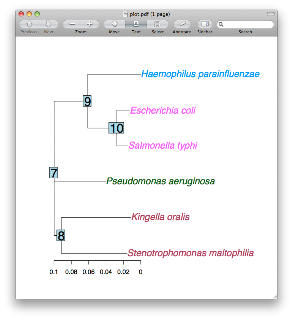I couldn't resist doing a little more with RPy and the R ape package. I used our standard tree file. The code listing is below. I am quite pleased with the results. With this in hand, it will be nice to look more at the ape book, which I already have.

In the first section we do our imports, and get the R object 'T' (True), because I couldn't get True or 'True' to work as a direct argument to the plot function. We also read the tree file. Next, I demonstrate the form of the ape function `drop_tip`, which is very useful, but I don't show results or a plot here.

In the third part, I define a function to return a list of colors based on the names of the external nodes, and then later convert that to an appropriate R object. This is what had me so frustrated when I first tried `ape`.

In the last part, we do the plot to a pdf file. The `x_lim` argument is important, that's how we stretch out the plot along the x-axis to make enough room for the names. Two separate calls after plot show the internal node labels, and the x-axis values.

I agree this is really powerful. The best part is that for someone like me, when you have trouble doing something in R, you can drop into Python without a problem. It's easy when you know how!

One more thing, Laurent Gautier is big in RPy, and one of his interests is Bioconductor. That project is the reason I got into R in the first place. It'll be fun to explore that in the future.

 `from rpy2 import robjectsfrom rpy2.robjects.packages import importrgrdevices = importr('grDevices')T = robjects.r['T']ape = importr('ape')fn = '/Users/telliott_admin/Desktop/tree.txt'tr = ape.read_tree(fn)print tr#--------------------------------------------# the ape function drop_tiptip_list = robjects.IntVector([1,6])#tr = ape.drop_tip(tr,tip_list)#--------------------------------------------# define some colors:# this works but isn't very flexible# col_list = robjects.r("rep(c('red','blue'),3)")def get_color(n): D = { 'Steno':'maroon','Kingella':'maroon', 'Haemo':'DodgerBlue', 'coli':'magenta','typhi':'magenta' } for k in D: if k in n: return D[k] return 'darkgreen'tip_labels = trcolor_list = [get_color(n) for n in tip_labels]color_list = robjects.StrVector(color_list)#--------------------------------------------ofn = '/Users/telliott_admin/Desktop/plot.pdf'grdevices.pdf(ofn)ape.plot_phylo(tr,tip_color=color_list, cex=1.5,x_lim=0.23)ape.nodelabels(cex=2)ape.axisPhylo()grdevices.dev_off()`